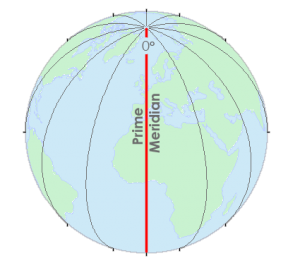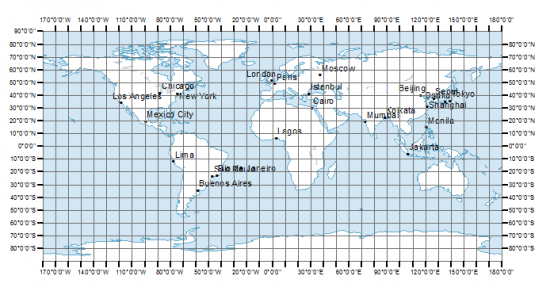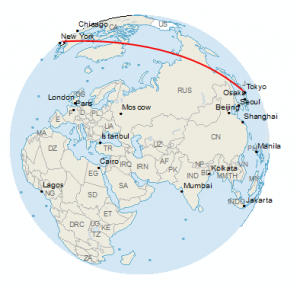# Latitude, Longitude and Coordinate System Grids

### Latitude, Longitude and Coordinate System Grids

Here’s how you can remember latitude and longitude:

Latitude lines run east-west and are parallel to each other. If you go north, latitude values increase. Finally, latitude values (Y-values) range between -90 and +90 degrees

But longitude lines run north-south. They converge at the poles. And its X-coordinates are between -180 and +180 degrees.

Latitude and longitude coordinates make up our geographic coordinate system.

### Map Coordinate Systems

You can give any location on Earth latitude and longitude coordinates.

The field of study that measures the shape and size of the Earth is geodesy. Geodesists use coordinate reference systems such as WGS84, NAD27 and NAD83. In each coordinate system, geodists use mathematics to give each position on Earth a unique coordinate.

A geographic coordinate system defines two-dimensional coordinates based on the Earth’s surface. It has an angular unit of measure, prime meridian and datum (which contains the spheroid).

As shown in the image below, lines of longitude have X-coordinates between -180 and +180 degrees.

And on the other hand, lines of latitudes have Y-values that are between -90 and +90 degrees.

The equator is where we measure north and south. For example, everything north of the equator has positive latitude values. Whereas, everything south of the equator has negative latitude values.

The Greenwich Meridian (or prime meridian) is a zero line of longitude from which we measure east and west. In fact, the zero line passes through the Royal Observatory in Greenwich, England, which is why we call it what it is today. In a geographical coordinate system, the prime meridian is the line that has 0° longitude.

Most horizontal datums assign the equator as a zero line of latitude. The equator is where we measure north and south. Whereas, the Greenwich Meridian (or prime meridian) is a zero line of longitude from which we measure east and west.Together, these lines provide a reference for latitude and longitude that always zig-zag into each other. This geographic grid gives unique latitude and longitude for every position on Earth.

### Find Anything on Earth with Coordinates

Coordinates are pairs (X, Y) in a two-dimensional space referenced to a horizontal datum. Whereas triplets (X, Y, Z) of points not only has position, but also has height referenced to a vertical datum. In other words, the X-value represents the horizontal position. Whereas, the Y-value represents the vertical position.Geographic coordinate systems use an ellipsoid to approximate all locations on the surface of the earth. Whereas, the datum defines the surface.

A horizontal datum has a major axis, which is the longest diameter of an ellipse. Also, it has a minor axis, which is the shortest diameter of an ellipse. Finally, a horizontal datum has a radius that represents the position of the surface relative to the center of the earth.

### What is a Coordinate Reference System?

A reference ellipsoid is the mathematical model of the shape of the Earth with the major axis along the equatorial radius. A geographic coordinate system uses longitude and latitude expressed in decimal degrees. For example, WGS 1984 and NAD 1983 are the most common datums today. Before 1983, NAD27 was the most common datum.Cartographers write spherical coordinates (latitudes and longitudes) in degrees-minutes-seconds (DMS) and decimal degrees. For degrees-minutes-seconds, minutes range from 0 to 60. For example, the geographic coordinate expressed in degrees-minutes-seconds for New York City is:

• Latitude: 40 degrees, 42 minutes, 51 seconds N
• Longitude: 74 degrees, 0 minutes, 21 seconds W

You can also express geographic coordinates in decimal degrees. It’s just another way to represent that same location in a different format. For example, here is New York City in decimal degrees:

• Latitude: 40.714
• Longitude: -74.006

The Federal Communications Commission has a DMS-Decimal converter tool that converts latitude and longitude between decimal degrees and degrees, minutes, and seconds.

### Latitude, Longitude and Spherical Coordinate System Grids

When you put two coordinates together as a pair (X, Y), you can locate anything on Earth.

Latitude and longitude form our coordinate system grid.

Also, you can express coordinates in different ways. For example, you can use decimal degrees or degrees-minutes-seconds.

Thanks to our geographic coordinate, you can pinpoint any point on Earth such as GPS receivers. And this includes the spot you are reading this article right now.

1. Allen Brock says:

Dennis Crane, if you still look here and haven’t had an answer about your Maryland coordinates, there was a program on the internet called “Corpscon7” which can convert coordinates like you have to regular LON LAT coordinates. It takes a little playing with, and a little knowledge, but it works great.

2. Robert B says:

In football, running North-South is to move down the length of the field ?!?

3. GISGeography says:

This is an interesting analogy! Thanks for the comment

4. William Pezzulich says:

Think in basic football terms.
A Lateral pass is a cross field pass Latitude North – South
Go Long is a downfield pass Longitude East – West

5. Jeferson Alandete says:

Interesting article. In some cases it’s required to incorporate a distance correction factors depending on your location and main reference point for the specific project,therefore, this correction factor can be bigger or smaller than 1 that can result in greater or smaller distances on the earth compare with the UTM grid.

In my current project we use what they’ve called Plant Coordinates which are UTM affected by the correction factor.

6. Chris Conohan says:

Is there a reason that latitude and longitude are given in that order instead of longitude and latitude? As I understand the map, it’s like using a coordinate pair of (y,x) instead of (x,y) which is what’s taught in school. Am I reading that incorrectly, or is there a reason for that convention?

7. GISGeography says:

Thanks for the comments on GISGeography, David! Very helpful for anyone learning these concepts.

8. David Verbyla says:

Think “ladder” for latitude (north south)
Long for longitude since longitude has a “longer” range of values up to 180 degrees, compare to up to 90 degrees for latitude

9. Dennis Crane says:

I have a similar question from Maryland. I have a plot plan that contains reference N: 429,433.02 and E: 1,455,456.46. This is an IPF location. Any suggestions?

10. Willard Handley says:

I have a set of property drawings of the public bus depot in Rochester, NY done in 1974. It shows the coordinates of the corners of the buildings on the property. The format of the coordinates is strange; for example, instead of the usual North and West, the first number is identified as “South,” the second as “East.”

For example: 49,957.44 South, 42,117.36 East. I thought they might be State Plane Coordinates, but I’ve confirmed that they are not. Ultimately I need to convert them into “normal” lat/long coordinates, but my question for now is just, what format is this?

11. Charles Benham says:

The longitudinal lines are perpendicular to the equator. Are the longitudes perpendicular to the latitudes?